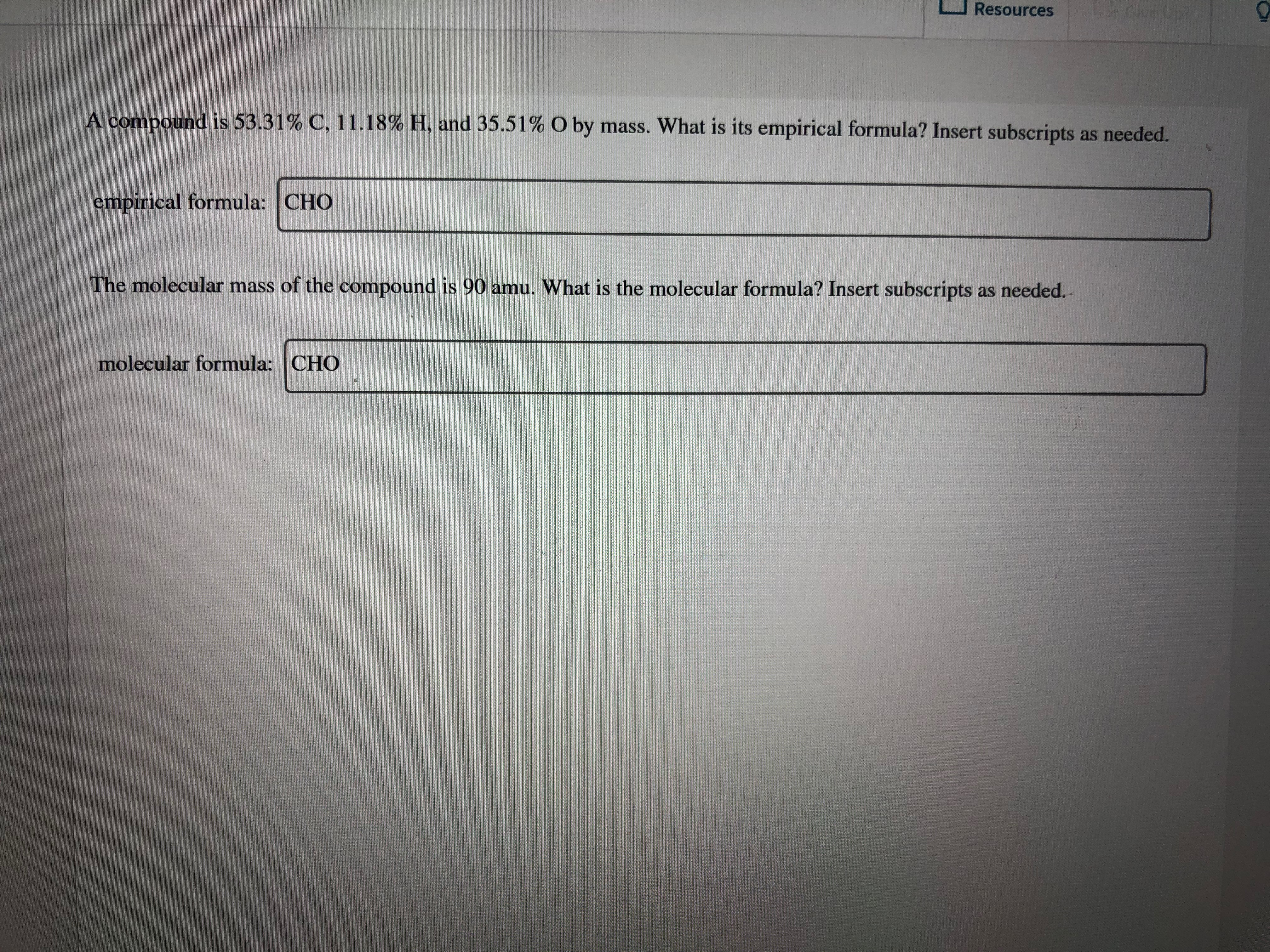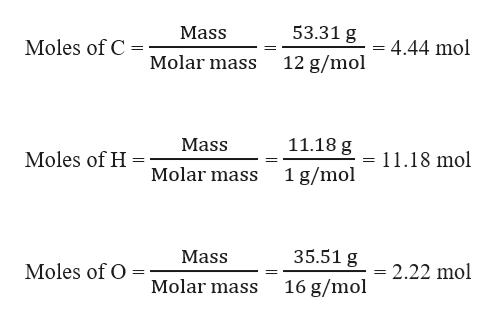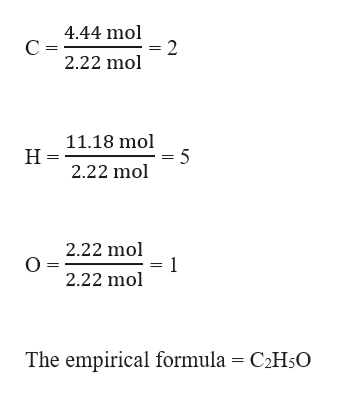# cive Lp?ResourcesA compound is 53.31% C, 11.18% H, and 35.51% O by mass. What is its empirical formula? Insert subscripts as needed.empirical formula: CHOThe molecular mass of the compound is 90 amu. What is the molecular formula? Insert subscripts as needed.molecular formula: CHO

Questionhelp_outlineImage Transcriptionclosecive Lp? Resources A compound is 53.31% C, 11.18% H, and 35.51% O by mass. What is its empirical formula? Insert subscripts as needed. empirical formula: CHO The molecular mass of the compound is 90 amu. What is the molecular formula? Insert subscripts as needed. molecular formula: CHO fullscreen
check_circleExpert Solution
Step 1

Given,

Mass percentage of C = 53.31 %

Mass percentage of H = 11.18 %

Mass percentage of O = 35.51 %

This means that 100 g of a compound contains 53.31 g of C, 11.18 g of H and 35.51 g of O.

Moles of C, H and O can be calculated as:help_outlineImage Transcriptionclose53.31 g Mass Moles of C 4.44 mol Molar mass 12 g/mol 11.18 g Mass Moles of H = 11.18 mol Molar mass 1 g/mol 35.51 g Mass Moles of O = 2.22 mol Molar mass 16 g/mol fullscreen
Step 2

The empirical formula can be given by calculating the simplest whole molar ratio as follows:help_outlineImage Transcriptionclose4.44 mol 2 2.22 mol С - 11.18 mol = 5 2.22 mol Н- 2.22 mol 1 2.22 mol The empirical formula = C2H50 fullscreen
Step 3

The molecular formula of a comp...

### Want to see the full answer?

See Solution

#### Want to see this answer and more?

Solutions are written by subject experts who are available 24/7. Questions are typically answered within 1 hour*

See Solution
*Response times may vary by subject and question
Tagged in

### General Chemistry